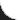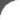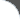Home | Tutorials | Articles | Videos | Products | Tools | Search
 Interviews | Open Source | Tag Cloud | Follow Us | Bookmark | ContactIn Browser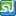StumbleUpon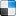del.icio.us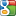Google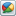Google Buzz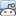reddit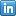LinkedIn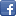Facebook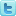TwitterLinkedin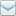E-Mail
 Design Patterns > Java Design Patterns > Factory Method

# Factory Method

Factory method comes under creational design pattern. It provides an abstraction to instantiate one of the available subclasses based on user input. It defers the class instantiation to subclasses.

• Lets the subclasses decide which class to instantiate.
• Connects parallel classes exhibiting the same behavior.
• We can parameterize factory method if needed.
• Decouples or hides concrete classes from client. That is if a particular implementation changes or given a new implementation all together, no need to change the client code.
• Gives fine control to provide a default implementation if the user requested implementation is not available.

In this example we have created a sort algorithm factory which instantiates one of the available sort algorithms based on user input. User doesn't need to know the actual implementation class. However we can hint the factory what type of algorithm we are expecting by passing a short name of algorithm.

Sort algorithm interface is shown below,

 File Name  : com/bethecoder/tutorials/dp/factory/ISortAlgorithm.java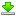``` package com.bethecoder.tutorials.dp.factory; public interface ISortAlgorithm {   /**    * Sorts the given list.    *     * @param list    */   public void sort(int [] list);    }```

Bubble sort implementation of sort algorithm.

 File Name  : com/bethecoder/tutorials/dp/factory/BubbleSort.java``` package com.bethecoder.tutorials.dp.factory; import java.util.Arrays; public class BubbleSort implements ISortAlgorithm {   @Override   public void sort(int[] list) {     //Dummy implementation     Arrays.sort(list);     System.out.println("Sorted using Bubble Sort Algorithm : " + Arrays.toString(list));   } }```

Merge sort implementation of sort algorithm.

 File Name  : com/bethecoder/tutorials/dp/factory/MergeSort.java``` package com.bethecoder.tutorials.dp.factory; import java.util.Arrays; public class MergeSort implements ISortAlgorithm {   @Override   public void sort(int[] list) {     //Dummy implementation     Arrays.sort(list);     System.out.println("Sorted using Merge Sort Algorithm : " + Arrays.toString(list));   } }```

Quick sort implementation of sort algorithm.

 File Name  : com/bethecoder/tutorials/dp/factory/QuickSort.java``` package com.bethecoder.tutorials.dp.factory; import java.util.Arrays; public class QuickSort implements ISortAlgorithm {   @Override   public void sort(int[] list) {     //Dummy implementation     Arrays.sort(list);     System.out.println("Sorted using Quick Sort Algorithm : " + Arrays.toString(list));   } }```

SortFactory internally maintains a static map, algorithmMap which contains short name for algorithm as key and implementation class as value. If we want to support other algorithms, we need to populate this map accordingly.

If the user provided algorithmName doesn't match with any available algorithms then we may choose to instantiate a default implementation of algorithm such as quick sort or throw an exception saying no such algorithm available. This enhancement is left as an exercise to readers.

The factory method implementation is shown below,

 File Name  : com/bethecoder/tutorials/dp/factory/SortFactory.java``` package com.bethecoder.tutorials.dp.factory; import java.util.HashMap; import java.util.Map; public class SortFactory {   private static Map> algorithmMap =      new HashMap>();      static {     algorithmMap.put("bubble", BubbleSort.class);     algorithmMap.put("merge", MergeSort.class);     algorithmMap.put("quick", QuickSort.class);   }      public static ISortAlgorithm getInstance(String algorithmName) {          Class algorithm = null;     ISortAlgorithm sortAlgorithmInstance = null;          if (algorithmName == null || !algorithmMap.containsKey(algorithmName.toLowerCase())) {       algorithm = QuickSort.class;     } else {       algorithm = algorithmMap.get(algorithmName.toLowerCase());     }          try {       sortAlgorithmInstance = algorithm.newInstance();     } catch (InstantiationException e) {       e.printStackTrace();     } catch (IllegalAccessException e) {       e.printStackTrace();     }          return sortAlgorithmInstance;   } }```

Here if we observe client code just deals with ISortAlgorithm and SortFactory. It doesn't need to know the actual implementation class. This decouples the client from actual implementation. So we can bring in more algorithms easily. Factory usage is shown below,

 File Name  : com/bethecoder/tutorials/dp/factory/Test.java``` package com.bethecoder.tutorials.dp.factory; public class Test {   /**    * @param args    */   public static void main(String[] args) {     int [] items  = {4, 3, 2, 1};     int [] items2 = {4, 3, 2, 1};     int [] items3 = {4, 3, 2, 1};          ISortAlgorithm sortAlgorithm = SortFactory.getInstance("bubble");     sortAlgorithm.sort(items);          sortAlgorithm = SortFactory.getInstance("quick");     sortAlgorithm.sort(items2);          sortAlgorithm = SortFactory.getInstance("merge");     sortAlgorithm.sort(items3);   } }```

It gives the following output,
```Sorted using Bubble Sort Algorithm : [1, 2, 3, 4]
Sorted using Quick Sort Algorithm : [1, 2, 3, 4]
Sorted using Merge Sort Algorithm : [1, 2, 3, 4]
```